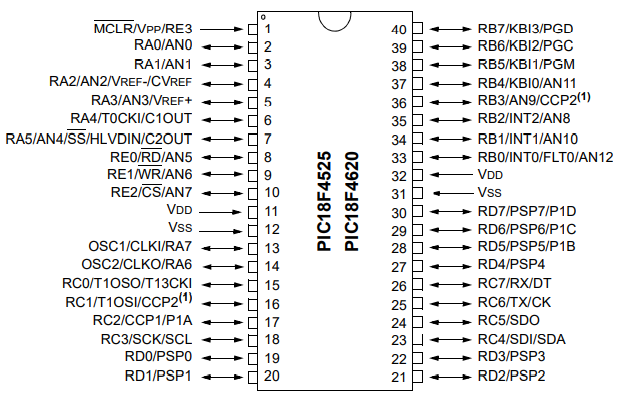﻿ How to Set the Ports of a PIC Microcontroller in C ﻿# How to Set the Ports of a PIC Microcontroller in CIn this article, we show how to set the ports of a PIC microcontroller in the C programming language.

The ports are the pins on a microcontroller that can control inputs or outputs to the microcontroller. Thus, the ports of a microcontroller are all bidirectional in that they can take an input voltage or emit an output voltage.

In reference to a specific microcontroller, the PIC18F4525 microcontroller that we are using, there are 5 ports, PORTA, PORTB, and PORTC.

The PIC18F4525 microcontroller is an 8-bit microcontroller. These means that each of the registers are made up of 8 bits, or pins.

This is shown in the schematic diagram below.PORTA is composed of the pins labelled RA0-RA7. PORTB is composed of pins labelled RB0-RB7. PORTC is composed of pin labelled RC0 to RC7. PORTD is composed of pins labelled RD0 to RD7. PORTE is composed of pins labelled RE0 to RE3.

When running a program on a microcontroller that uses inputs and outputs, the port(s) being used must be set in code. This way, we can set which pins will be used as inputs and which pins will be used as outputs.

So setting the pins of a port of a microcontroller can be done using binary values, hexadecimal values, and decimal values.

Remember that the microcontroller we are using is an 8-bit microcontroller, so each PORT (register) has 8 bits that need to be set.

To set the pins of a port, you must use a special function register called TRIS to do so. There is a TRIS for each PORT, and each TRIS has the same number of bits as each PORT. As you set the numerical value of TRIS from left to right, you are setting the bits in order of greatest to least: RA7, RA6, RA5, RA4, RA3, RA2, RA1, RA0.

TRIS controls whether each pin in a PORT is an input or an output.

If the programmer gives a '1' to the bit, then the bit of the PORT is an input.

If the programmer gives a '0' to the bit, then the bit of the PORT is an output.

### Setting a PORT in Binary

So we will first show how to set a PORT in binary values.

We will set the bits on PORTA and PORTB.

We will make the first 2 bits of PORTA as inputs and the next 6 bits as outputs.

We will make the first 5 bits of PORTB as outputs and the last 3 bit as inputs.

The code to do this is shown below.

Remember that the first bits appear to the rightmost, since the most significant bit (RA7 or RB7) is leftmost.

As another example, to make all bits in PORTA inputs and all bits in PORTB outputs, we use the following code below.

Now all bits in PORTA are inputs and all bits in PORTB are outputs.

### Setting a PORT in Hexadecimal

Now we show how to set a PORT in hexadecimal values.

The advantage of setting a PORT with hexadecimal values is that the number used is shorter than if using other number formats.

The disadvantage is that many times it requires calculation or some type of converter to know the value that must be used, but with practice, a programmer may know what codes correspond to what

We will set the bits on PORTA and PORTB.

We will make the first 2 bits of PORTA as inputs and the next 6 bits as outputs.

We will make the first 5 bits of PORTB as outputs and the last 3 bit as inputs.

The code to do this is shown below.

To make all bits in PORTA inputs and all bits in PORTB outputs, we use the following code below.

Now all bits in PORTA are inputs and all bits in PORTB are outputs.

### Setting a PORT in Decimal

Lastly we show how to set a PORT in decimal values.

Just like the other examples, we will set the bits on PORTA and PORTB.

We will make the first 2 bits of PORTA as inputs and the next 6 bits as outputs.

We will make the first 5 bits of PORTB as outputs and the last 3 bit as inputs.

The code to do this is shown below.

To make all bits in PORTA inputs and all bits in PORTB outputs, we use the following code below.

Now all bits in PORTA are inputs and all bits in PORTB are outputs.

Notice that unlike binary and hexadecimal, no prefix is used with decimal, because decimal is the default radix for MPLABX, which is the software we use to run this code. When no prefix is used, the software labels it as a decimal value.

And this is how to set the ports of a PIC microcontroller as inputs or outputs in the C programming language.

Related Resources

How to Enable or Disable the Watchdog Timer of a PIC Microcontroller in C